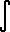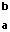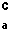# Math2.org Math Tables: Integral Identities(Math)

Formal Integral Definition:when...

a = x0 < x1 < x2 < ... < xn = b
d = max (x1-x0, x2-x1, ... , xn - x(n-1))
xk-1 <= Xk <= xk     k = 1, 2, ... , nF '(x) dx = F(b) - F(a) (Fundamental Theorem for integrals of derivatives)a f(x) dx = af(x) dx (if a is constant)f(x) + g(x) dx =f(x) dx +g(x) dx

$\int_a^b f(x) dx = \left[ \int f(x) \, dx \right]_a^b$f(x) dx +f(x) dx =f(x) dx(integration by substitution)